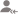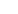true

Take Class 10 Tuition from the Best Tutors

•Affordable fees
•1-1 or Group class
•Flexible Timings
•Verified Tutors

Search in# Learn UNIT V: Trigonometry with Free Lessons & Tips

Post a Lesson

All

All

Lessons

Discussion

2. A bridge across the river makes an angle of 45 with the river bank. If the length of the bridge across... read more
2. A bridge across the river makes an angle of 45° with the river bank. If the length of the bridge across the river is 150 m, what is the width of the river? read lessJaisna

Maths online tuition for class 5 to 12.

Dislike Bookmark

3. There is a small island in the middle of a 100 m wide river and a tall tree stands on the island.... read more
3. There is a small island in the middle of a 100 m wide river and a tall tree stands on the island. P and Q are the points directly opposite to each other on the two banks, and in a line with the tree. If the angles of elevation of the top of the tree from P and Q are 30° and 45° respectively, find the height of the tree. read lessJaisna

Maths online tuition for class 5 to 12.

Dislike Bookmark

6. A tower subtends an angle at a point A in the place of its base and the angle of depression of the... read more
6. A tower subtends an angle α at a point A in the place of its base and the angle of depression of the foot of the tower at a point b ft. just above A is β. Prove that the height of the tower is b tan α cot β. read lessJaisna

Maths online tuition for class 5 to 12.

Let CD is the height of the tower. ie, C is the bottom of the tower. Let AB is b ft. in trinagle ABC, (1) In triangle ACD, (2) From (1) and (2)/ therefore, read more

Let CD is the height of the tower. ie, C is the bottom of the tower.

Let AB is b ft.

in trinagle ABC,

(1)

In triangle ACD,

(2)

From (1) and (2)/

therefore,

Dislike Bookmark

Take Class 10 Tuition from the Best Tutors

•Affordable fees
•Flexible Timings
•Choose between 1-1 and Group class
•Verified Tutors

9. In a right triangle ABC, right-angled at B, if tan A = 1, then verify that 2 sin A cos A = 1.Shailesh Kaushik

Contact me @9899486175 if needed

Tan A = 1 means A is 45 degrees. which means cosA = cos45 = 1/√2 and sinA = sin45 = 1/√2 therefore, 2sinAcosA = 2 * 1/√2 * 1/√2 = 2 * (1/2) = 1 read more

Tan A = 1 means A is 45 degrees.

which means cosA = cos45 = 1/√2 and sinA = sin45 = 1/√2

therefore, 2sinAcosA = 2 * 1/√2 * 1/√2

= 2 * (1/2)

= 1

Dislike Bookmark

5. A flagstaff stands at the top of a 5m high tower. From a point on the ground, the angle of elevation... read more
5. A flagstaff stands at the top of a 5m high tower. From a point on the ground, the angle of elevation of the top of the flagstaff is 60° and from the same point, the angle of elevation of the top of the tower is 45°. Find the height of the flagstaff. read less

7. A vertical tower stands on a horizontal plane and is surmounted by a flagstaff of height 7m. From... read more
7. A vertical tower stands on a horizontal plane and is surmounted by a flagstaff of height 7m. From a point on the plane, the angle of elevation of the bottom of the flagstaff is 30° and that of the top of the flagstaff is 45°. Find the height of the tower. read less

Take Class 10 Tuition from the Best Tutors

•Affordable fees
•Flexible Timings
•Choose between 1-1 and Group class
•Verified Tutors

5. Find the value of sin 45 °- Cos 45°.

6. If 4 tan θ = 3, evaluate (4 sin θ – cos θ + 1)/(4 sin θ + cos θ + 1).

7. Prove that [tan θ/(1 – cot θ)] + [cot θ/(1 – tan θ)] = 1 + sec θ cosec θ

Take Class 10 Tuition from the Best Tutors

•Affordable fees
•Flexible Timings
•Choose between 1-1 and Group class
•Verified Tutors

8. Prove that: sin θ/(cot θ + cosec θ) = 2 + [sin θ/ (cot θ – cosec θ]

UrbanPro.com helps you to connect with the best Class 10 Tuition in India. Post Your Requirement today and get connected.

Overview

Questions 22

Total Shares1 Followers

## Class 10 Tuition in:

x

X

### Looking for Class 10 Tuition Classes?

The best tutors for Class 10 Tuition Classes are on UrbanPro

• Select the best Tutor
• Book & Attend a Free Demo
• Pay and start Learning### Take Class 10 Tuition with the Best Tutors

The best Tutors for Class 10 Tuition Classes are on UrbanProUrbanPro.com is India's largest network of most trusted tutors and institutes. Over 55 lakh students rely on UrbanPro.com, to fulfill their learning requirements across 1,000+ categories. Using UrbanPro.com, parents, and students can compare multiple Tutors and Institutes and choose the one that best suits their requirements. More than 7.5 lakh verified Tutors and Institutes are helping millions of students every day and growing their tutoring business on UrbanPro.com. Whether you are looking for a tutor to learn mathematics, a German language trainer to brush up your German language skills or an institute to upgrade your IT skills, we have got the best selection of Tutors and Training Institutes for you. Read more